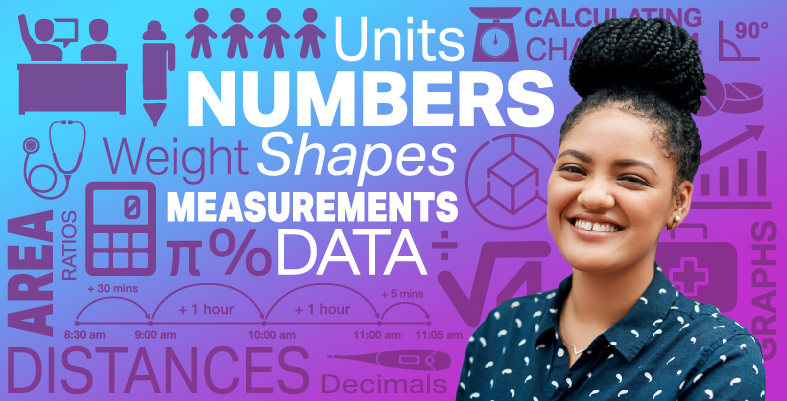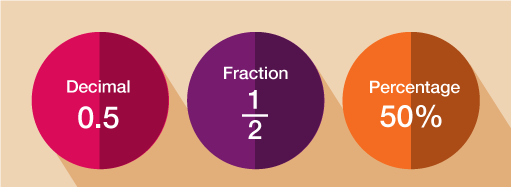Everyday maths for Health and Social Care and Education Support 2

Start this free course now. Just create an account and sign in. Enrol and complete the course for a free statement of participation or digital badge if available.

Free course

# 6 Fractions, decimals and percentages

You have already worked with decimals in this course and many times throughout your life. Every time you calculate something to do with money, you are using decimal numbers. You have also learned how to round a number to a given number of decimal places.Figure 8 Equivalent decimals, fractions and percentages

Since fractions, decimals and percentages are all just different ways of representing the same thing, we can convert between them in order to compare. Take a look at the video below to see how to convert fractions, decimals and percentages.Skip transcript

#### Transcript

Equivalent fractions, decimals, and percentages are all just different ways of representing the same thing, so you can convert between them to compare. First, let's look at turning percentages into decimals. Take the example of 60%. Remember that a percentage is out of 100. To turn a percentage into its equivalent decimal, you need to divide by 100. 60 divided by 100 = 0.6. So 0.6 is the equivalent decimal of 60%.

What about 25%? Can you work out the equivalent decimal of 25% before the answer is revealed? Remember that 'cent' means 100.

To turn 25% into its equivalent decimal, you need to divide 25 by 100, which = 0.25.

Now let's find the equivalent fraction for the decimals we've calculated. To do this, you need to first turn it into a percentage and write it as a fraction out of 100. Using our previous examples, 0.6 or 60%, can be written as 60 over 100. 0.25, or 25%, can be written as 25 out of 100.

However, these fractions are not written in their simplest form. Remember that to simplify fractions, you need to divide both parts by the same number and keep going until you can't find a number that you can divide both parts by. In their simplest form, 0.6 is written as the fraction 3/5. 0.25 is written as the fraction one quarter, or 1 over 4.

Now looking at the table, you can see how percentages, decimals and fractions relate to each other.

Let's try one more example. Can you work out the equivalent decimal and fraction of 72%? 72 divided by 100 gives the decimal 0.72. To find the equivalent fraction, you need to change it into a percentage and then write it as a fraction out of 100. Then simplify the fraction into its simplest form, 18 out of 25, 18/25.

End transcript

Interactive feature not available in single page view (see it in standard view).

## Activity 12: Matching fractions, decimals and percentages

Choose the correct fraction for each percentage and decimal.

Using the following two lists, match each numbered item with the correct letter.

• a.      62.5% = 0.625 =

• b.      40% = 0.4 =

• c.      50% = 0.5 =

• d.      35% = 0.35 =

• 1 = d
• 2 = b
• 3 = c
• 4 = a

Fractions and percentages deal with splitting numbers into a given number of equal portions, or parts. When dealing with the next topic, ratio, you will still be splitting quantities into a given number of parts, but when sharing in a ratio, you do not share evenly. This might sound a little complicated but you’ll have been doing it since you were a child.

## Summary

In this section you have:

• learned about the relationship between fractions, decimals and percentages and are now able to convert between the three.

FSM_SSH_2